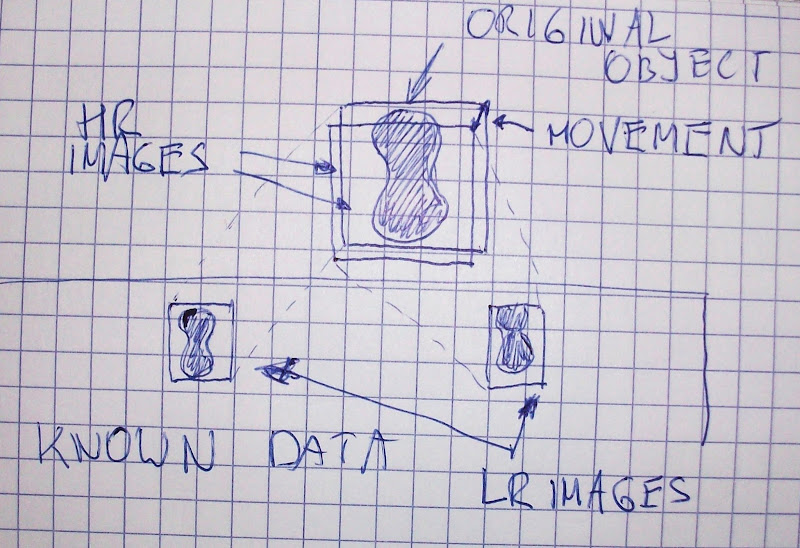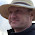### Abstract

One of important steps in super resolution algorithms is the estimation of initial constants, without this the algorithms will produce useless higher resolution images.

From mathematical view, we may say that during taking a photo, the original scenery is gathered in high resolution, with some movement between each image (e.g. shaking hand or satellite moving on its orbit), next psf and the noise is added, at the end the image is downscaled.To obtain higher resolution we need to somehow revert this process, to do that we need to estimate mentioned movements. In this post I will present two simple algorithms that I used to estimate the movement between images.

### First attempt

It wasn't successful, in about 50% cases answers were incorrect, but still better this, than nothing.

Input:
• A and B - input, low resolution images.
• n - downscale factor used to produce A and B from the original, high resolution scenery.
Algorithm:
• upscale images n times.
• Select randomly a lot of points on A, for each point:
• find the offset where the difference between (x and its neighbors) and its corresponding pixels on the second image is smallest, if it's impossible, then ignore this point.
• calculate the movement (x,y) as a ratio between sum of all estimations from 1. 2. and total amount of checked points.
Output:
• (x,y) is the movement between original images that were used to create A and B images.

The implementation is full of hardcoded constants, I didn't clean it up because I moved to a new algorithm..

### Second attempt

Input:
• A and B - input, low resolution images.
• n - downscale factor used to produce A and B from the original, high resolution scenery.
Algorithm:
• upscale images n times, convert it to black and white (two colors only).
• Select a point in the upper left corner and move step-by-step into the bottom right corner until you have a pixel that has more that three neighbors with different color, then:
• find the offset where the difference between (x and its neighbors) and its corresponding pixels on the second image is smallest, if it's impossible, then ignore this point.
• calculate the movement (x,y) as a ratio between sum of all estimations from 1. 2. and total amount of checked points.
Output:
• (x,y) is the movement between original images that were used to create A and B images.

Results are very good, answers are correct in about 75-100% cases. The new implementation has even more weird constants, but I will clean it up soon, so don't forget to watch the project :D

### Conclusions

At the beginning I was thinking that motion estimation will be a very difficult task in this case, but it's not that hard. It seems that the second algorithm is good enough and it works fine with the rest of supper resolution scripts.

#### 1 comment:

1.I had a question on super resolution and trying to align images that were taken using a fisheye lens. I found your work on github referenced from some similar questions to mine, and was wondering if you had any suggestions.
http://dsp.stackexchange.com/questions/14602/motion-and-distortion-estimation-using-multiple-still-images
Great scripts btw, thank you!